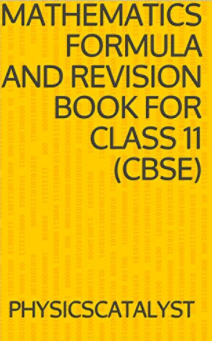Home » Maths » Class 11 Maths Formula book

# Class 11 Maths Formula book

## Class 11 Maths Formula bookThis is a Mathematics formula and revision book for class 11 (CBSE). Having all the formula in one place is always helpful for the students, Keeping that in mind,We have prepared a Maths formulas pdf for CBSE Class 11 students.  This math formula book contains the maths formulas that are aimed for class 11 students as per the NCERT math book .You can also use it as a revision guide for competitive exams like JEE. This book covers formula list and summary for following topics

1. Algebra of sets
2. Relations and Functions
3. Trigonometric Functions
4. Mathematical induction
5. Complex algebra
6. Quadratic equations and complex roots
7. Inequalities
8. Permutation and combination
9. Binomial theorem
10. Series and sequences
11. Coordinate geometry and Straight line
12. Conic sections
13. Three-dimensional Coordinate geometry
14. Limits and derivatives
15. Statistics
16. Probability